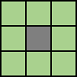# bwlabel

Label connected components in 2-D binary image

## Syntax

``L = bwlabel(BW)``
``L = bwlabel(BW,conn)``
``````[L,n] = bwlabel(___)``````

## Description

example

````L = bwlabel(BW)` returns the label matrix `L` that contains labels for the 8-connected objects found in `BW`.```
````L = bwlabel(BW,conn)` returns a label matrix, where `conn` specifies the connectivity.```
``````[L,n] = bwlabel(___)``` also returns `n`, the number of connected objects found in `BW`.```

## Examples

collapse all

Create a small binary image.

```BW = logical ([1 1 1 0 0 0 0 0 1 1 1 0 1 1 0 0 1 1 1 0 1 1 0 0 1 1 1 0 0 0 1 0 1 1 1 0 0 0 1 0 1 1 1 0 0 0 1 0 1 1 1 0 0 1 1 0 1 1 1 0 0 0 0 0]);```

Create the label matrix using 4-connected objects.

`L = bwlabel(BW,4)`
```L = 8×8 1 1 1 0 0 0 0 0 1 1 1 0 2 2 0 0 1 1 1 0 2 2 0 0 1 1 1 0 0 0 3 0 1 1 1 0 0 0 3 0 1 1 1 0 0 0 3 0 1 1 1 0 0 3 3 0 1 1 1 0 0 0 0 0 ```

Use the `find` command to get the row and column coordinates of the object labeled "2".

```[r, c] = find(L==2); rc = [r c]```
```rc = 4×2 2 5 3 5 2 6 3 6 ```

## Input Arguments

collapse all

Binary image, specified as a 2-D numeric matrix or 2-D logical matrix. For numeric input, any nonzero pixels are considered to be `1` (`true`).

Data Types: `single` | `double` | `int8` | `int16` | `int32` | `int64` | `uint8` | `uint16` | `uint32` | `uint64` | `logical`

Pixel connectivity, specified as one of these values.

Value

Meaning

Two-Dimensional Connectivities

`4`

Pixels are connected if their edges touch. Two adjoining pixels are part of the same object if they are both on and are connected along the horizontal or vertical direction.Current pixel is shown in gray.

`8`

Pixels are connected if their edges or corners touch. Two adjoining pixels are part of the same object if they are both on and are connected along the horizontal, vertical, or diagonal direction.Current pixel is shown in gray.

Data Types: `double` | `logical`

## Output Arguments

collapse all

Label matrix of contiguous regions, returned as matrix of nonnegative integers with the same size as `BW`. The pixels labeled `0` are the background. The pixels labeled `1` make up one object; the pixels labeled `2` make up a second object; and so on.

Data Types: `double`

Number of connected objects in `BW`, returned as a nonnegative integer.

Data Types: `double`

## Tips

• The functions `bwlabel`, `bwlabeln`, and `bwconncomp` all compute connected components for binary images. `bwconncomp` replaces the use of `bwlabel` and `bwlabeln`. It uses significantly less memory and is sometimes faster than the other functions.

Input DimensionOutput FormMemory UseConnectivity
`bwlabel`2-DDouble-precision label matrixHigh4 or 8
`bwlabeln`N-DDouble-precision label matrixHighAny
`bwconncomp`N-DCC structLowAny
• You can use the MATLAB® `find` function in conjunction with `bwlabel` to return vectors of indices for the pixels that make up a specific object. For example, to return the coordinates for the pixels in object 2, enter the following:.

`[r,c] = find(bwlabel(BW)==2)`

You can display the output matrix as a pseudocolor indexed image. Each object appears in a different color, so the objects are easier to distinguish than in the original image. For more information, see `label2rgb`.

• To extract features from a binary image using `regionprops` with default connectivity, just pass `BW` directly into `regionprops` using the command `regionprops(BW)`.

• The `bwlabel` function can take advantage of hardware optimization for data types `logical`, `uint8`, and `single` to run faster. Hardware optimization requires `marker` and `mask` to be 2-D images and `conn` to be either 4 or 8.

## Algorithms

`bwlabel` uses the general procedure outlined in reference , pp. 40-48:

1. Run-length encode the input image.

2. Scan the runs, assigning preliminary labels and recording label equivalences in a local equivalence table.

3. Resolve the equivalence classes.

4. Relabel the runs based on the resolved equivalence classes.

 Haralick, Robert M., and Linda G. Shapiro, Computer and Robot Vision, Volume I, Addison-Wesley, 1992, pp. 28-48.